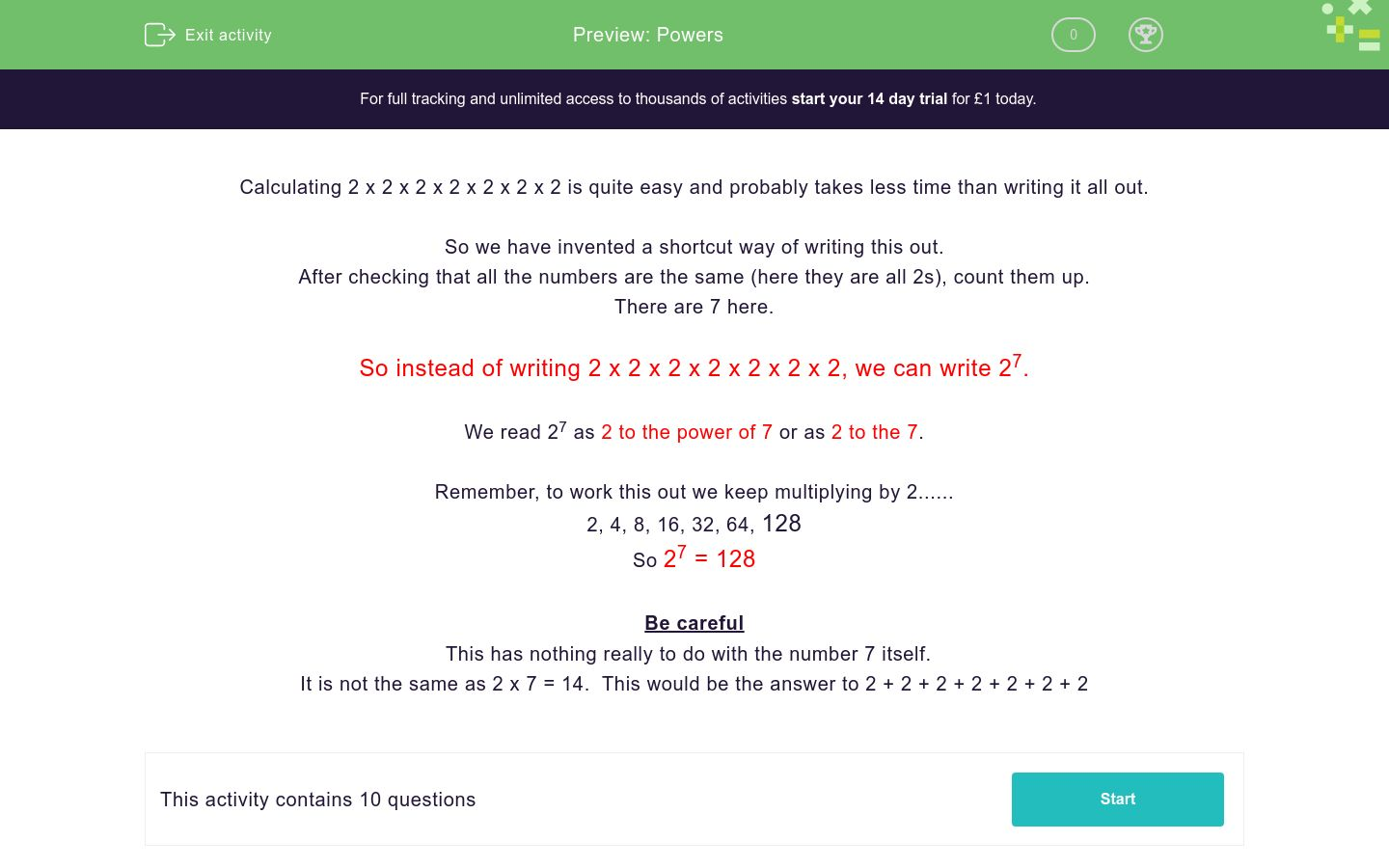# Powers

In this worksheet, students simplify numerical expressions using powers.Key stage:  KS 3

Curriculum topic:   Number

Curriculum subtopic:   Understand Integer Powers/Real Roots

Difficulty level:### QUESTION 1 of 10

Calculating 2 x 2 x 2 x 2 x 2 x 2 x 2 is quite easy and probably takes less time than writing it all out.

So we have invented a shortcut way of writing this out.

After checking that all the numbers are the same (here they are all 2s), count them up.

There are 7 here.

So instead of writing 2 x 2 x 2 x 2 x 2 x 2 x 2, we can write 27.

We read 27 as 2 to the power of 7 or as 2 to the 7.

Remember, to work this out we keep multiplying by 2......

2, 4, 8, 16, 32, 64, 128

So 27 = 128

Be careful

This has nothing really to do with the number 7 itself.

It is not the same as 2 x 7 = 14.  This would be the answer to 2 + 2 + 2 + 2 + 2 + 2 + 2

Select all the answers which are equal to:

2 x 2 x 2 x 2

8

2 x 4

16

24

Select all the answers which are equal to:

2 x 2 x 2 x 2 x 2

32

2 x 5

10

25

Select all the answers which are equal to:

3 x 3 x 3

33

12

33

27

Select all the answers which are equal to:

3 x 3 x 3 x 3

81

9 x 9

34

12

Select all the answers which are equal to:

3 x 3 x 3 x 3 x 3

53

35

343

243

Select all the answers which are equal to:

10 x 10 x 10

103

310

30

1000

Select all the answers which are equal to:

10 x 10 x 10 x 10 x 10 x 10

106

a million

60

1000000

Select all the answers which are equal to:

5 x 5 x 5 x 5

45

54

625

20

Select all the answers which are equal to:

1 x 1 x 1 x 1 x 1 x 1

16

61

111111

1

Select all the answers which are equal to:

2 x 2 x 2 x 2 x 2 x 2 x 2

128

27

2 x 7

14

• Question 1

Select all the answers which are equal to:

2 x 2 x 2 x 2

16
24
EDDIE SAYS
Working out 2 x 2 x 2 x 2 would give 16 There are four number twos multiplied together, we can write this as 24
• Question 2

Select all the answers which are equal to:

2 x 2 x 2 x 2 x 2

32
25
EDDIE SAYS
Working out 2 x 2 x 2 x 2 x 2 would give 32 There are five number twos multiplied together, we can write this as 25
• Question 3

Select all the answers which are equal to:

3 x 3 x 3

33
27
EDDIE SAYS
Working out 3 x 3 x 3 would give 27 There are three number threes multiplied together, we can write this as 33
• Question 4

Select all the answers which are equal to:

3 x 3 x 3 x 3

81
9 x 9
34
EDDIE SAYS
Working out 3 x3 x 3 x 3 would give 81 There are four number threes multiplied together, we can write this as 34
• Question 5

Select all the answers which are equal to:

3 x 3 x 3 x 3 x 3

35
243
EDDIE SAYS
Working out 3 x3 x 3 x 3 x 3 would give 243 There are five number threes multiplied together, we can write this as 35
• Question 6

Select all the answers which are equal to:

10 x 10 x 10

103
1000
EDDIE SAYS
Working out 10 x 10 x 10 would give 1000 There are three number tens multiplied together, we can write this as 103
• Question 7

Select all the answers which are equal to:

10 x 10 x 10 x 10 x 10 x 10

106
a million
1000000
EDDIE SAYS
Working out 10 x 10 x 10 x 10 x 10 x 10 would give 1000000 This can be written in words as A Million There are six number tens multiplied together, we can write this as 106
• Question 8

Select all the answers which are equal to:

5 x 5 x 5 x 5

54
625
EDDIE SAYS
Working out 5 x 5 x5 x 5 would give 625 There are four number fives multiplied together, we can write this as 54
• Question 9

Select all the answers which are equal to:

1 x 1 x 1 x 1 x 1 x 1

16
1
EDDIE SAYS
Working out 1 x 1 x 1 x 1 x 1 x 1 would give 1 There are six number ones multiplied together, we can write this as 16
• Question 10

Select all the answers which are equal to:

2 x 2 x 2 x 2 x 2 x 2 x 2

128
27
EDDIE SAYS
Working out 2 x 2 x 2 x 2 x 2 x 2 x 2 would give 128 There are seven number twos multiplied together, we can write this as 27
---- OR ----

Sign up for a £1 trial so you can track and measure your child's progress on this activity.

### What is EdPlace?

We're your National Curriculum aligned online education content provider helping each child succeed in English, maths and science from year 1 to GCSE. With an EdPlace account you’ll be able to track and measure progress, helping each child achieve their best. We build confidence and attainment by personalising each child’s learning at a level that suits them.

Get started